### Merry N'Mas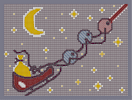Hover over the thumbnail for a full-size version.

Author the_happy_taco author:the_happy_taco bitesized christmas n-art nonplayable rated 2007-12-26 2007-12-26 5 by 54 people. \$Merry N'Mas#the_happy_taco#none#00000000000000000000000000000000000000000000000000000000000000000000000000000000000000000000000000000000000000000000000000000000000000000000000000000000000000000000000000000000000000000000000000000000000000000000000000000000000000000000000000000000000000000000000000000000000000000000000000000000000000000000000000000000000000000000000000000000000000000000000000000000000000000000000000000000000000000000000000000000000000000000000000000000000000000000000000000000000000000000000000000000000000000000000000000000000000000000000000000000000000000000000000000000000000000000000000000000000000000000000000000000000000000000000000000000000000000000000000000000000000000000000000000000000000000000000000000000000000000|10^84,438!10^318,444!10^144,546!10^294,402!12^319,439!12^319,437!12^319,435!12^319,430!12^319,424!12^318,418!12^315,412!12^313,409!12^306,403!12^301,400!12^300,400!12^81,442!12^81,444!12^81,447!12^81,452!12^81,459!12^81,464!12^81,469!12^83,479!12^83,489!12^85,498!12^85,505!12^85,508!12^86,511!12^87,516!12^89,524!12^96,530!12^102,536!12^105,540!12^113,545!12^120,546!12^128,546!12^133,546!12^136,546!12^85,490!12^84,486!12^84,484!12^84,480!12^82,478!12^82,475!10^151,575!10^157,573!10^165,569!10^169,565!10^175,560!10^182,558!10^189,553!10^191,551!10^197,547!10^206,541!10^211,538!10^219,534!10^223,531!10^230,528!10^237,525!10^243,521!10^250,517!10^256,513!10^263,508!10^272,503!10^277,499!10^283,494!10^289,490!10^298,485!10^306,479!10^316,474!10^323,468!10^332,461!10^338,456!10^311,477!10^302,482!10^293,489!10^270,505!10^201,544!10^186,556!10^176,559!10^261,511!10^327,465!10^334,458!10^319,470!10^288,492!10^281,495!10^247,518!10^241,522!10^234,527!10^228,530!10^341,452!10^343,452!10^346,448!10^348,446!10^353,443!10^355,440!10^358,436!10^360,431!10^361,428!10^362,426!10^364,422!10^364,417!10^364,413!10^364,409!10^87,440!10^93,442!10^101,448!10^105,452!10^108,453!10^112,457!10^121,459!10^122,459!10^130,460!10^135,461!10^141,464!10^145,464!10^147,464!10^158,464!10^160,464!10^173,464!10^173,464!10^166,464!10^175,465!10^184,465!10^185,465!10^191,465!10^193,465!10^198,463!10^200,462!10^203,462!10^211,459!10^213,459!10^219,457!10^224,456!10^227,454!10^234,454!10^237,452!10^271,434!10^274,429!10^280,423!10^283,421!10^284,417!10^285,415!10^287,411!10^287,411!10^292,405!10^240,451!10^243,451!10^247,450!10^250,449!10^256,444!10^260,442!10^264,437!10^265,436!10^96,444!10^116,363!10^122,363!10^124,363!10^127,364!10^128,364!10^134,363!10^134,362!10^135,365!0^120,354!0^126,354!0^120,372!0^126,372!0^114,380!0^114,383!0^109,390!0^109,391!0^103,399!0^99,403!0^93,410!0^90,417!0^90,420!0^90,426!0^90,431!0^91,431!0^106,396!0^132,378!0^132,379!0^132,382!0^136,386!0^138,389!0^144,393!0^147,394!0^154,398!0^159,401!0^166,405!0^168,409!0^173,417!0^177,428!0^178,430!0^178,433!0^179,435!0^179,439!0^180,443!0^180,444!0^182,449!0^182,457!0^182,457!0^175,425!0^175,420!0^172,416!0^170,413!10^116,362!10^118,363!10^121,363!10^124,363!10^126,363!10^128,363!10^130,363!10^133,363!10^133,363!10^114,363!10^111,363!10^106,361!10^105,358!10^104,358!10^102,357!10^97,353!10^97,353!10^109,363!10^108,364!10^105,366!10^101,366!10^98,369!10^96,372!10^94,372!10^100,353!10^94,351!10^93,375!10^91,375!0^131,347!0^133,341!0^135,339!0^138,335!0^138,335!0^132,333!0^129,334!0^123,334!0^121,334!0^113,333!0^113,334!0^110,335!0^111,340!0^112,340!0^183,453!0^120,378!0^126,378!0^126,384!0^120,384!0^120,390!0^114,390!0^114,396!0^108,396!0^114,402!0^108,402!0^102,402!0^102,408!0^96,408!0^96,414!0^102,414!0^108,414!0^108,408!0^126,348!0^120,348!0^114,342!0^120,342!0^126,342!0^114,348!0^132,336!0^126,336!0^120,336!0^114,336!0^126,390!0^132,390!0^132,396!0^126,396!0^120,396!0^120,402!0^114,408!0^114,414!0^120,414!0^120,408!0^126,408!0^126,402!0^126,402!0^132,402!0^132,408!0^132,414!0^126,414!0^126,420!0^120,420!0^114,420!0^108,420!0^102,420!0^96,420!0^96,426!0^96,432!0^132,384!0^138,396!0^138,402!0^138,414!0^138,408!0^144,396!0^150,402!0^144,402!0^144,408!0^150,408!0^150,414!0^144,414!0^144,420!0^138,420!0^132,420!0^132,426!0^126,426!0^120,426!0^114,426!0^108,426!0^102,426!0^102,432!0^102,438!0^96,438!0^108,432!0^114,432!0^126,432!0^120,432!0^120,438!0^114,438!0^108,438!0^108,444!0^114,444!0^102,444!0^90,438!0^150,396!0^156,402!0^162,408!0^168,414!0^168,420!0^174,426!0^174,432!0^174,438!0^174,444!0^174,450!0^174,456!0^180,456!0^180,450!0^156,408!0^156,414!0^162,414!0^162,420!0^156,420!0^150,420!0^150,426!0^144,426!0^138,426!0^138,432!0^132,432!0^138,438!0^132,438!0^126,438!0^126,444!0^120,444!0^120,450!0^114,450!0^126,450!0^132,450!0^132,444!0^138,444!0^138,450!0^138,456!0^132,456!0^144,456!0^150,456!0^156,456!0^162,456!0^168,456!0^162,450!0^168,450!0^168,444!0^168,444!0^168,438!0^168,432!0^168,426!0^162,426!0^156,426!0^156,432!0^162,432!0^162,438!0^162,444!0^156,444!0^156,450!0^150,450!0^144,450!0^144,444!0^144,438!0^144,432!0^150,432!0^150,438!0^150,444!0^156,438!10^82,434!10^89,438!10^89,441!10^91,442!10^93,444!10^94,446!10^100,448!10^100,448!10^102,450!10^105,452!10^106,452!10^107,456!10^111,456!10^117,458!10^118,458!10^122,461!10^131,463!10^131,463!10^138,464!10^140,464!10^145,465!10^145,465!10^155,467!10^157,467!10^159,467!10^165,467!10^170,467!10^175,467!10^180,467!10^183,467!10^185,467!10^194,464!10^197,464!10^200,464!10^202,464!10^207,462!10^209,460!10^216,460!10^216,458!10^218,458!10^224,457!10^230,457!10^232,454!10^240,454!10^252,449!10^256,447!10^257,444!10^258,439!10^263,437!10^264,434!10^268,430!10^272,428!10^274,425!10^279,421!10^283,417!10^283,413!10^285,411!10^286,409!10^290,405!10^291,404!10^82,434!10^81,436!10^83,437!10^83,437!10^154,573!10^160,573!10^162,567!10^165,567!10^169,563!10^170,562!10^176,557!10^182,557!10^188,555!10^190,553!10^195,550!10^197,547!10^200,545!10^202,544!10^205,544!10^209,542!10^212,538!10^213,537!10^215,534!10^220,534!10^222,531!10^229,527!10^236,526!10^242,521!10^249,519!10^256,514!10^260,513!10^264,509!10^270,506!10^272,503!10^278,499!10^286,495!10^294,485!10^297,483!10^308,481!10^310,478!10^319,474!10^321,472!10^325,470!10^328,467!10^335,461!10^338,457!10^339,453!10^332,463!10^350,447!10^351,445!10^355,442!10^356,440!10^357,434!10^361,430!10^363,423!10^363,419!10^363,414!10^364,408!10^271,504!12^310,405!12^84,494!12^88,519!12^124,546!12^118,546!12^108,542!12^92,529!12^94,532!12^97,533!0^114,378!0^133,378!0^129,376!0^118,377!0^117,372!0^129,373!0^118,354!0^130,353!0^117,340!0^124,344!0^124,349!0^129,337!0^129,342!0^125,350!10^432,300!10^426,300!10^420,300!10^414,300!10^408,300!10^402,300!10^396,306!10^390,312!10^384,318!10^378,324!10^378,330!10^378,336!10^378,342!10^378,348!10^378,354!10^378,360!10^384,366!10^390,372!10^396,378!10^402,384!10^408,384!10^414,384!10^420,384!10^426,384!10^432,384!10^438,384!10^444,378!10^450,372!10^456,366!10^462,360!10^462,354!10^462,348!10^462,342!10^462,336!10^462,330!10^462,324!10^456,318!10^450,312!10^444,306!10^438,300!10^492,264!10^486,258!10^480,252!10^474,246!10^468,240!10^498,264!10^504,264!10^510,264!10^516,264!10^522,264!10^528,264!10^468,234!10^468,228!10^468,222!10^468,216!10^468,210!10^468,204!10^534,258!10^540,252!10^546,246!10^552,240!10^552,234!10^552,228!10^552,222!10^552,216!10^552,210!10^552,204!10^546,198!10^534,186!10^528,180!10^522,180!10^516,180!10^510,180!10^504,180!10^498,180!10^492,180!10^486,186!10^480,192!10^612,198!10^606,192!10^600,186!10^594,180!10^588,174!10^618,198!10^630,198!10^624,198!10^636,198!10^642,198!10^648,198!10^654,192!10^660,186!10^666,180!10^672,174!10^672,168!10^672,162!10^672,156!10^672,150!10^672,144!10^672,138!10^666,132!10^660,126!10^654,120!10^648,114!10^642,114!10^636,114!10^630,114!10^624,114!10^618,114!10^612,114!10^606,120!10^600,126!10^594,132!10^588,138!10^588,144!10^588,150!10^588,156!10^588,162!10^588,168!10^148,544!10^153,540!10^154,540!10^202,515!10^209,512!10^212,511!10^213,509!10^218,509!10^221,506!10^226,505!10^230,502!10^231,500!10^235,499!10^238,496!10^316,445!10^308,451!10^308,453!10^302,456!10^298,456!10^295,459!10^291,462!10^286,467!10^283,468!10^278,471!10^274,472!10^268,478!10^264,481!10^258,485!10^251,489!10^243,494!10^247,492!10^252,490!10^197,516!10^191,519!10^186,522!10^183,525!10^181,525!10^176,528!10^172,531!10^168,533!10^164,534!10^158,540!10^160,536!10^259,487!10^144,546!10^151,542!10^154,539!10^158,537!10^162,534!10^165,533!10^170,531!10^175,528!10^182,525!10^190,524!10^194,519!10^195,519!10^204,513!10^204,510!10^183,523!10^183,524!10^191,520!10^195,516!10^201,516!10^204,515!10^210,511!10^213,509!10^219,506!10^222,504!10^226,502!10^228,501!10^231,499!10^236,496!10^244,491!10^245,490!10^247,489!10^252,487!10^257,486!10^262,483!10^265,480!10^267,480!10^272,476!10^275,472!10^278,469!10^281,466!10^283,465!10^285,463!10^289,462!10^291,460!10^295,458!10^299,457!10^305,454!10^307,453!10^309,451!10^311,449!10^313,447!10^313,447!10^186,531!10^186,531!10^186,533!10^188,535!10^189,536!10^192,541!10^192,542!10^195,537!10^195,535!10^194,534!10^190,531!10^188,529!10^188,527!10^274,477!10^274,477!10^276,479!10^282,485!10^282,486!10^280,486!10^277,485!10^281,476!10^280,477!10^286,482!10^286,482!10^286,486!10^284,483!10^280,480!10^277,476!12^84,450!12^84,456!12^84,462!12^84,468!12^84,474!12^90,480!12^90,474!12^90,468!12^90,462!12^90,456!12^90,450!12^90,486!12^90,492!12^90,498!12^90,504!12^90,510!12^90,516!12^90,522!12^96,528!12^96,522!12^96,522!12^96,516!12^96,510!12^96,504!12^96,492!12^96,486!12^96,480!12^96,474!12^96,498!12^84,498!12^96,468!12^96,462!12^96,456!12^96,450!12^84,444!12^102,534!12^102,528!12^102,522!12^102,516!12^102,516!12^102,510!12^108,510!12^144,498!12^150,498!12^156,510!12^162,510!12^162,504!12^162,498!12^162,492!12^162,486!12^168,486!12^174,486!12^180,486!12^192,486!12^186,486!12^198,486!12^204,486!12^204,480!12^210,480!12^216,480!12^216,486!12^210,486!12^210,492!12^210,498!12^210,504!12^204,504!12^198,504!12^198,498!12^198,486!12^204,492!12^198,492!12^204,498!12^192,492!12^186,492!12^180,492!12^174,492!12^168,492!12^168,498!12^168,504!12^168,510!12^174,510!12^180,510!12^186,510!12^192,510!12^198,510!12^186,516!12^180,516!12^174,522!12^168,522!12^168,522!12^168,516!12^174,516!12^174,504!12^174,498!12^180,498!12^186,498!12^186,498!12^192,498!12^192,504!12^186,504!12^180,504!12^102,456!12^102,462!12^102,468!12^102,474!12^102,480!12^102,486!12^102,492!12^102,498!12^102,504!12^162,528!12^156,528!12^168,528!12^162,522!12^162,516!12^156,516!12^114,516!12^108,516!12^108,528!12^108,522!12^108,534!12^108,540!12^114,540!12^120,540!12^126,540!12^132,540!12^138,540!12^138,534!12^144,540!12^144,534!12^150,534!12^150,528!12^156,522!12^120,522!12^114,522!12^114,534!12^114,528!12^120,528!12^126,528!12^132,534!12^126,534!12^120,534!12^156,504!12^156,498!12^108,504!12^132,492!12^108,492!12^108,498!12^114,486!12^120,480!12^126,486!12^108,486!12^108,480!12^108,474!12^108,468!12^108,462!12^114,468!12^114,474!12^114,480!12^114,462!12^138,492!12^144,492!12^150,492!12^156,492!12^156,486!12^156,480!12^150,480!12^144,480!12^138,480!12^132,480!12^126,480!12^150,486!12^144,486!12^138,486!12^132,486!12^120,468!12^120,462!12^120,468!12^120,474!12^126,468!12^126,474!12^132,468!12^132,474!12^138,468!12^138,474!12^144,474!12^150,474!12^156,474!12^162,474!12^168,474!12^174,474!12^180,474!12^186,474!12^192,474!12^198,474!12^204,474!12^210,474!12^216,474!12^204,468!12^216,468!12^210,468!12^198,468!12^156,480!12^162,480!12^168,480!12^174,480!12^180,480!12^186,480!12^192,480!12^198,480!12^216,492!12^216,498!12^222,498!12^222,492!12^222,486!12^222,480!12^222,468!12^222,474!12^222,462!12^216,504!12^300,408!12^306,408!12^306,414!12^312,414!12^312,426!12^306,420!12^312,420!12^312,432!12^312,438!12^306,438!12^306,444!12^300,444!12^300,438!12^300,432!12^306,432!12^306,426!12^300,426!12^300,420!12^300,414!12^294,414!12^294,420!12^294,426!12^294,432!12^294,438!12^294,444!12^294,450!12^300,450!12^288,450!12^288,444!12^288,438!12^288,432!12^288,426!12^288,420!12^282,426!12^282,432!12^282,438!12^282,444!12^282,450!12^282,456!12^288,456!12^276,456!12^276,450!12^276,444!12^276,444!12^276,438!12^276,432!12^270,438!12^270,444!12^270,450!12^270,456!12^276,462!12^270,462!12^270,468!12^264,468!12^258,474!12^264,474!12^258,480!12^252,480!12^228,492!12^234,492!12^240,486!12^234,486!12^246,480!12^240,480!12^234,480!12^228,480!12^228,486!12^228,474!12^228,468!12^228,462!12^234,462!12^240,462!12^246,462!12^252,462!12^258,462!12^264,462!12^264,456!12^264,450!12^264,444!12^258,456!12^258,450!12^252,456!12^246,456!12^240,456!12^258,468!12^252,468!12^246,468!12^240,468!12^234,468!12^234,474!12^240,474!12^252,474!12^246,474!12^150,504!12^132,528!12^126,522!12^120,516!12^114,510!12^138,528!12^144,528!12^120,486!12^612,120!12^624,120!12^636,120!12^648,120!12^642,126!12^630,126!12^618,126!12^606,126!12^612,132!12^600,132!12^606,138!12^600,144!12^612,144!12^606,150!12^600,156!12^612,156!12^606,162!12^600,168!12^612,168!12^606,174!12^612,180!12^600,180!12^606,186!12^618,186!12^612,192!12^624,192!12^636,192!12^648,192!12^642,186!12^630,186!12^618,174!12^624,180!12^624,168!12^618,162!12^624,156!12^618,150!12^624,144!12^618,138!12^624,132!12^630,138!12^636,132!12^654,126!12^660,132!12^666,138!12^666,150!12^666,162!12^666,174!12^654,174!12^660,180!12^660,168!12^660,156!12^660,144!12^654,150!12^654,162!12^654,186!12^648,180!12^594,138!12^594,150!12^594,162!12^594,174!12^636,144!12^630,150!12^642,150!12^648,156!12^636,156!12^630,162!12^642,162!12^648,168!12^642,174!12^636,168!12^630,174!12^636,180!10^147,547!10^153,543!10^156,539!10^161,537!10^163,535!10^167,534!10^170,533!10^172,532!10^175,531!10^178,528!10^181,526!10^186,524!10^190,522!10^194,522!10^198,519!10^200,515!10^203,514!10^208,514!10^211,510!10^216,509!10^219,508!10^223,506!10^225,505!10^229,502!10^233,498!10^236,497!10^241,494!10^244,492!10^248,491!10^251,489!10^255,487!10^259,485!10^263,483!10^267,479!10^268,479!10^272,475!10^278,471!10^282,468!10^289,460!10^292,459!10^296,456!10^299,455!10^304,452!10^307,451!10^310,451!10^312,449!10^315,448!10^315,448!10^317,445!10^261,485!10^83,436!10^87,441!10^91,442!10^92,442!10^96,447!10^97,449!10^99,450!10^103,451!10^107,453!10^109,456!10^114,456!10^115,456!10^118,457!10^119,457!10^120,457!10^121,459!10^123,459!10^124,459!10^131,461!10^133,462!10^136,462!10^138,462!10^149,466!10^152,466!10^155,466!10^158,466!10^162,466!10^166,466!10^171,466!10^173,466!10^181,466!10^183,466!10^188,466!10^193,466!10^198,464!10^206,460!10^211,460!10^215,458!10^218,458!10^219,456!10^225,456!10^233,456!10^235,453!10^238,450!10^240,449!10^240,449!10^247,444!10^257,438!10^265,429!10^273,426!10^278,423!10^278,420!10^281,418!10^284,415!10^284,412!10^286,410!10^287,407!10^288,405!10^290,403!10^290,403!10^249,443!10^252,443!10^299,399!10^303,400!10^308,400!10^311,402!10^314,407!10^316,411!10^317,413!10^318,415!10^318,417!10^318,421!10^318,427!10^318,432!10^318,438!10^318,444!10^315,446!10^319,440!10^319,440!10^319,437!10^319,434!10^319,431!10^319,425!10^319,420!10^318,414!10^314,408!10^312,405!10^310,403!10^308,403!10^304,401!10^303,401!10^299,401!10^296,401!10^295,402!10^293,403!10^79,442!10^79,445!10^79,448!10^79,450!10^79,453!10^79,457!10^79,460!10^79,464!10^79,467!10^79,467!10^79,471!10^79,472!10^79,477!10^79,484!10^79,485!10^79,489!10^79,492!10^79,495!10^79,499!10^79,503!10^80,509!10^80,511!10^81,514!10^81,516!10^83,518!10^83,522!10^87,526!10^87,527!10^141,547!10^139,547!10^134,547!10^130,548!10^126,548!10^124,548!10^120,547!10^117,547!10^115,546!10^112,544!10^107,543!10^105,540!10^102,537!10^96,535!10^93,533!10^88,528!10^85,523!10^167,567!10^171,565!10^176,561!10^182,557!10^184,555!10^189,553!10^192,550!10^198,548!10^199,547!10^204,543!10^207,540!10^208,540!10^214,538!10^217,535!10^219,534!10^220,533!10^225,532!10^230,530!10^236,525!10^238,523!10^240,521!10^243,520!10^246,520!10^251,519!10^256,516!10^259,513!10^260,513!10^262,511!10^264,508!10^266,507!10^270,507!10^271,505!10^274,502!10^276,500!10^280,497!10^283,493!10^286,491!10^290,488!10^292,486!10^300,482!10^305,479!10^307,477!10^311,476!10^311,473!10^316,469!10^321,466!10^325,463!10^331,460!10^335,458!10^474,198!10^612,114!10^606,120!10^594,132!10^588,138!10^588,144!10^588,150!10^588,156!10^588,162!10^588,168!10^588,174!10^594,180!10^600,186!10^606,192!10^612,198!10^618,198!10^624,198!10^630,198!10^636,198!10^642,198!10^648,198!10^654,192!10^660,186!10^666,180!10^672,174!10^672,168!10^672,162!10^672,156!10^672,150!10^672,144!10^672,138!10^672,138!10^666,132!10^660,126!10^654,120!11^474,588,492,186!11^498,588,510,186!11^522,588,528,186!11^546,588,498,192!11^570,588,516,192!11^594,588,486,198!11^618,588,480,192!11^642,588,504,198!11^660,588,534,192!11^684,588,522,198!11^708,588,540,198!11^732,588,474,204!11^756,588,492,204!11^450,588,510,204!11^420,588,528,204!11^396,588,546,204!11^366,588,480,210!11^342,588,498,210!11^780,588,516,210!11^318,588,534,210!11^294,588,540,216!11^270,588,522,216!11^246,588,504,216!11^222,588,486,216!11^198,588,468,216!11^174,588,546,222!11^150,588,528,222!11^126,588,510,222!11^102,588,492,222!11^78,588,474,222!11^54,588,480,228!11^30,588,498,228!11^6,588,516,228!11^384,588,534,228!11^432,588,540,234!11^510,588,522,234!11^534,588,504,234!11^534,588,486,234!11^546,588,468,234!11^552,588,474,240!11^534,588,492,240!11^534,588,480,246!11^480,588,486,252!11^492,588,498,246!11^486,588,510,240!11^468,588,516,246!11^516,588,504,252!11^468,588,492,258!11^504,588,510,258!11^414,588,528,240!11^432,588,522,252!11^432,588,534,246!11^486,588,546,240!11^492,588,528,258!11^546,588,540,252!10^528,180!10^522,180!10^516,180!10^510,180!10^504,180!10^498,180!10^492,180!10^486,186!10^480,192!10^474,198!10^468,204!10^468,216!10^468,210!10^468,222!10^468,228!10^468,234!10^468,240!10^474,246!10^480,252!10^486,258!10^498,264!10^510,264!10^516,264!10^522,264!10^528,264!10^534,258!10^540,252!10^546,246!10^552,240!10^552,234!10^552,228!10^552,222!10^552,216!10^552,210!10^552,204!10^546,198!10^546,198!10^534,186!11^492,588,402,306!11^438,588,420,306!11^408,588,438,306!11^366,588,408,312!11^432,588,390,312!11^402,588,426,312!11^426,588,444,312!11^444,588,432,318!11^432,588,414,318!11^402,588,420,324!11^420,588,426,330!11^426,588,432,336!11^426,588,438,342!11^438,588,444,348!11^438,588,450,354!11^396,588,396,318!11^414,588,402,324!11^426,588,408,330!11^426,588,414,336!11^426,588,420,342!11^396,588,426,348!11^516,588,432,354!11^450,588,438,360!11^492,588,456,360!11^474,588,444,366!11^456,588,438,324!11^444,588,444,330!11^444,588,450,336!11^462,588,456,342!11^396,588,450,318!11^468,588,456,324!11^456,588,384,324!11^390,588,390,330!11^390,588,396,336!11^396,588,402,342!11^384,588,408,348!11^414,588,414,354!11^420,588,420,360!11^402,588,426,366!11^444,588,432,372!11^408,588,450,372!11^420,588,438,378!11^408,588,384,342!11^396,588,390,348!11^396,588,396,354!11^432,588,384,360!11^432,588,390,366!11^414,588,402,360!11^414,588,408,366!11^444,588,414,372!11^426,588,420,378!11^438,588,396,372!11^426,588,402,378!10^438,300!10^432,300!10^426,300!10^420,300!10^414,300!10^408,300!10^402,300!10^396,306!10^390,312!10^384,318!10^378,324!10^378,336!10^378,342!10^378,354!10^378,360!10^384,366!10^390,372!10^396,378!10^402,384!10^408,384!10^414,384!10^420,384!10^426,384!10^432,384!10^438,384!10^444,378!10^450,372!10^456,366!10^462,360!10^462,354!10^462,342!10^462,336!10^462,324!10^456,318!10^444,306!10^450,312!10^539,191!10^527,201!10^527,201!10^525,202!10^522,206!10^522,207!10^522,211!10^527,212!10^528,212!10^530,212!10^533,211!10^534,206!10^534,202!10^531,201!10^527,202!10^527,209!10^527,210!10^530,208!10^531,206!10^531,206!10^439,322!10^438,320!10^436,319!10^432,319!10^430,321!10^430,328!10^435,331!10^439,331!10^440,331!10^440,328!10^439,325!10^435,325!10^434,327!10^438,328!10^439,328!10^436,325!10^433,321!10^434,322!10^437,324!10^442,325!10^442,324!10^442,323!10^651,135!10^646,135!10^643,136!10^643,144!10^644,146!10^646,146!10^650,144!10^651,140!10^650,137!10^646,138!10^647,143!10^651,140!10^648,137!10^645,141!10^651,139!10^645,134!10^645,141!10^645,141!10^644,141!10^644,138!10^644,137!10^648,137!10^648,137!10^648,143!10^648,146!10^648,146!10^645,146!10^644,146!10^642,142!10^642,140!10^642,137!10^649,137!10^649,137!10^651,137!10^651,139!10^651,140!10^653,140!10^246,438!10^240,432!10^234,426!10^228,420!10^222,414!10^234,414!10^216,408!10^210,402!10^204,396!10^186,414!10^198,402!10^192,408!10^180,420!10^187,420!10^194,418!10^201,416!10^206,415!10^210,414!10^205,406!10^202,407!10^201,407!10^201,408!10^239,408!10^241,402!10^244,397!10^253,400!10^253,403!10^247,403!10^245,407!10^241,398!10^257,401!10^262,401!10^264,400!10^268,398!10^273,397!10^274,396!10^281,391!10^288,390!10^297,390!10^302,389!10^306,388!10^310,388!10^313,386!10^316,386!10^320,386!10^322,386!10^325,389!10^327,389!10^332,390!10^335,390!10^340,390!10^346,392!10^349,392!10^351,392!10^355,393!10^356,393!10^364,394!10^370,394!10^377,394!10^384,394!10^390,394!10^395,394!10^401,392!10^404,390!10^409,388!10^413,384!10^416,377!10^417,376!10^417,370!10^417,369!10^417,365!10^417,359!10^417,355!10^417,353!10^417,351!10^418,351!10^421,351!10^424,354!10^430,354!10^437,354!10^439,354!10^447,354!10^456,354!10^462,354!10^470,354!10^475,354!10^480,354!10^488,349!10^497,343!10^505,337!10^510,332!10^513,329!10^514,325!10^518,320!10^518,317!10^518,311!10^518,306!10^518,299!10^518,293!10^518,285!10^518,275!10^518,263!10^518,253!10^518,249!10^515,240!10^515,240!10^515,240!10^517,249!10^517,258!10^517,268!10^517,276!10^517,277!10^518,238!10^521,245!10^529,250!10^535,254!10^543,258!10^551,262!10^557,262!10^564,262!10^573,262!10^581,262!10^587,262!10^593,262!10^597,262!10^610,257!10^615,255!10^623,251!10^628,248!10^630,242!10^631,236!10^631,228!10^604,260!10^607,260!10^631,222!10^631,220!10^631,218!10^631,211!10^631,204!10^631,199!10^631,193!10^631,185!10^631,178!10^631,174!10^631,174!10^631,174!10^631,174!10^631,177!10^514,233!10^514,234!10^514,234!10^512,234!10^512,234!10^417,350!10^279,391!10^295,387!10^309,384!10^313,383!10^316,383!10^329,380!10^333,380!10^337,378!10^343,374!10^343,373!10^348,372!10^351,371!10^357,367!10^361,365!10^364,363!10^368,362!10^372,361!10^457,311!10^462,311!10^467,312!10^473,312!10^476,307!10^479,304!10^483,300!10^488,297!10^492,292!10^494,287!10^496,282!10^496,277!10^496,273!10^496,270!10^559,222!10^561,222!10^565,222!10^569,222!10^575,222!10^577,220!10^588,217!10^594,215!10^600,208!10^600,206!10^604,202!10^604,200!10^583,218!10^286,389!10^291,389!10^193,415!10^193,413!10^224,419!10^220,420!10^220,423!10^217,426!10^213,429!10^211,433!10^208,431!10^206,431!10^203,432!10^199,435!10^199,439!10^201,440!10^202,441!10^205,441!10^207,439!10^207,437!10^199,430!10^199,430!10^196,425!10^187,405!10^185,402!10^180,396!10^177,393!10^173,388!10^171,381!10^171,370!10^171,363!10^171,354!10^172,348!10^173,338!10^172,330!10^167,323!10^158,315!10^152,311!10^151,310!10^163,318!10^171,327!10^171,331!10^171,340!10^171,345!10^171,348!10^171,354!10^172,359!10^172,362!10^172,371!10^172,372!10^172,375!10^172,377!10^172,377!10^172,378!10^147,306!10^146,306!10^145,306!10^142,306!10^138,307!10^136,308!10^132,309!10^129,311!10^128,312!10^122,314!10^122,314!10^119,314!10^115,314!10^112,314!10^109,315!10^106,315!10^98,315!10^94,312!10^90,309!10^87,305!10^83,302!10^203,399!10^203,399!10^202,401!10^198,401!10^196,402!10^196,404!10^193,407!10^191,409!10^189,410!10^188,410!10^186,411!10^183,414!10^183,416!10^183,420!10^194,417!10^197,417!10^198,417!10^200,417!10^202,416!10^204,414!10^213,414!10^213,414!10^205,396!10^205,396!10^207,397!10^210,399!10^211,401!10^212,403!10^213,404!10^215,406!10^217,411!10^217,412!10^219,413!10^220,414!10^221,414!10^223,415!10^227,416!10^227,418!10^230,422!10^230,423!10^232,425!10^232,426!10^236,429!10^237,430!10^238,433!10^244,437!10^246,438!10^247,438!10^250,441!10^251,442!10^242,409!10^238,411!10^234,413!10^234,415!10^232,417!10^231,418!10^229,419!10^227,422!10^219,423!10^215,430!10^211,432!10^210,432!10^207,434!10^204,435!10^201,438!10^201,438!10^244,406!10^244,406!10^244,399!10^243,399!10^241,399!10^239,402!10^239,402!10^180,420!10^180,420!10^181,419!0^230,57!0^224,58!0^210,64!0^201,68!0^192,78!0^180,95!0^176,114!0^176,131!0^176,155!0^190,180!0^206,194!0^222,199!0^230,199!0^233,199!0^233,60!0^229,62!0^214,73!0^207,81!0^198,122!0^198,143!0^198,166!0^214,183!0^228,188!0^224,66!0^219,70!0^211,77!0^204,83!0^202,87!0^199,130!0^199,136!0^199,144!0^200,153!0^200,164!0^206,176!0^213,184!0^200,147!0^200,156!0^200,161!0^202,164!0^204,172!0^210,178!0^216,181!0^221,184!0^226,188!0^229,188!0^238,190!0^243,191!0^247,191!0^247,190!0^235,189!0^250,192!0^247,193!0^242,197!0^239,199!0^224,199!0^218,200!0^213,200!0^209,196!0^202,192!0^198,188!0^191,182!0^188,179!0^183,168!0^178,161!0^177,155!0^176,146!0^179,166!0^185,174!0^214,199!0^202,196!0^200,194!0^196,189!0^192,185!0^185,175!0^179,165!0^209,199!0^228,59!0^221,59!0^214,59!0^211,60!0^207,62!0^203,64!0^197,68!0^193,70!0^188,73!0^177,83!0^176,94!0^175,101!0^175,110!0^175,117!0^189,77!0^180,82!0^178,87!0^172,93!0^170,104!0^170,112!0^167,125!0^167,137!0^167,148!0^168,160!0^171,175!0^180,181!0^183,185!0^193,192!0^202,197!0^216,66!0^210,66!0^222,66!0^210,72!0^204,72!0^204,78!0^198,78!0^198,72!0^192,72!0^192,78!0^186,78!0^186,84!0^198,84!0^204,66!0^192,84!0^198,90!0^192,90!0^186,90!0^180,90!0^174,90!0^186,78!0^186,78!0^180,78!0^180,174!0^174,168!0^174,162!0^174,150!0^174,144!0^174,132!0^174,126!0^174,120!0^174,114!0^186,96!0^192,96!0^192,96!0^192,102!0^186,102!0^186,102!0^180,102!0^198,126!0^198,138!0^198,144!0^198,156!0^198,162!0^192,108!0^186,108!0^180,108!0^174,108!0^168,108!0^174,96!0^186,114!0^180,114!0^192,114!0^192,120!0^192,126!0^192,132!0^192,138!0^192,144!0^192,150!0^192,156!0^192,162!0^198,150!0^198,168!0^204,168!0^204,174!0^204,180!0^210,186!0^240,192!0^234,192!0^228,192!0^222,192!0^216,192!0^216,198!0^222,186!0^216,186!0^204,186!0^210,192!0^204,192!0^210,180!0^198,180!0^192,180!0^192,186!0^198,186!0^186,186!0^174,96!0^168,114!0^180,120!0^186,120!0^186,126!0^180,126!0^180,132!0^186,132!0^198,132!0^174,138!0^180,138!0^186,138!0^186,144!0^180,144!0^180,156!0^180,150!0^186,150!0^186,156!0^186,162!0^180,162!0^186,168!0^192,168!0^192,174!0^198,174!0^180,174!0^180,168!0^186,180!0^174,174!0^174,180!0^168,96!0^168,120!0^168,132!0^162,132!0^156,132!0^168,102!0^162,102!0^162,114!0^162,126!0^162,120!0^162,108!0^168,144!0^168,150!0^168,156!0^174,156!0^168,162!0^168,168!0^168,174!0^162,101!0^159,103!0^156,106!0^154,109!0^150,114!0^149,124!0^149,134!0^149,146!0^152,158!0^161,170!0^165,175!0^151,108!0^153,103!0^158,98!0^169,91!0^181,81!0^192,75!0^162,108!0^168,96!0^162,96!0^156,114!0^156,120!0^150,120!0^156,126!0^150,126!0^150,132!0^162,138!0^156,138!0^162,90!0^174,84!0^168,84!0^174,78!0^180,72!0^186,66!0^186,72!0^240,54!0^234,60!0^234,54!0^228,54!0^222,54!0^216,54!0^210,60!0^204,60!0^198,66!0^192,66!0^198,60!0^246,198!0^234,204!0^222,204!0^228,204!0^216,204!0^210,204!0^204,198!0^198,198!0^192,198!0^186,192!0^180,192!0^186,198!0^180,186!0^174,186!0^162,162!0^162,156!0^162,150!0^162,144!0^156,144!0^150,138!0^150,150!0^150,144!0^156,150!0^156,156!0^150,156!0^156,162!0^162,168!0^156,168!0^168,180!0^168,138!0^168,126!0^174,102!0^156,108!0^180,84!0^180,78!0^162,102!0^156,102!0^198,192!0^192,78!0^192,72!0^180,96!0^186,174!0^168,174!0^234,198!0^228,198!0^222,198!0^216,198!0^240,198!0^252,192!0^252,198!0^258,192!0^210,198!0^204,204!0^198,96!0^198,102!0^198,108!0^198,114!0^198,120!0^612,312!0^612,324!0^612,318!0^612,330!0^612,336!0^606,324!0^600,324!0^618,324!0^624,324!0^612,54!0^612,60!0^612,66!0^606,60!0^618,60!0^468,480!0^468,492!0^468,486!0^468,498!0^468,504!0^462,492!0^456,492!0^474,492!0^480,492!0^708,228!0^708,234!0^708,240!0^708,246!0^708,252!0^708,258!0^708,264!0^708,270!0^708,276!0^714,252!0^720,252!0^726,252!0^732,252!0^702,252!0^696,252!0^690,252!0^684,252!0^702,246!0^714,246!0^714,258!0^702,258!0^48,540!0^612,228!12^654,114!12^660,120!12^666,126!12^666,120!12^660,114!12^666,114!12^666,108!12^672,114!12^672,108!12^678,108!12^672,102!12^678,102!12^684,102!12^678,96!12^684,96!12^690,96!12^684,90!12^690,90!12^696,90!12^690,84!12^696,84!12^702,84!12^696,78!12^702,78!12^708,78!12^702,72!12^708,72!12^714,72!12^708,66!12^714,66!12^720,66!12^714,60!12^720,60!12^726,60!12^720,54!12^726,54!12^732,54!12^726,48!12^732,48!12^738,48!12^732,42!12^738,42!12^744,42!12^738,36!12^744,36!12^750,36!12^744,30!12^750,30!12^756,30!12^750,24!12^756,24!10^672,138!10^666,132!10^660,126!10^654,120!10^648,114!10^768,576!10^24,24!10^48,24!10^72,24!10^96,24!10^120,24!10^144,24!10^168,24!10^192,24!10^216,24!10^240,24!10^264,24!10^288,24!10^312,24!10^336,24!10^360,24!10^384,24!10^408,24!10^432,24!10^456,24!10^480,24!10^504,24!10^528,24!10^552,24!10^576,24!10^600,24!10^624,24!10^648,24!10^672,24!10^696,24!10^720,24!10^36,36!10^36,60!10^36,84!10^36,108!10^36,132!10^36,156!10^36,180!10^36,204!10^36,228!10^36,252!10^36,276!10^36,300!10^60,300!10^60,348!10^60,324!10^48,336!10^48,312!10^24,312!10^24,336!10^84,36!10^84,60!0^84,84!10^84,108!10^84,132!0^84,180!0^84,174!0^90,180!0^78,180!0^84,186!0^36,324!0^36,318!0^36,330!0^42,324!0^30,324!10^108,36!10^96,48!10^96,72!10^96,96!10^72,72!10^72,96!10^48,96!10^60,108!10^60,84!10^60,60!10^72,48!10^60,36!10^48,48!10^48,72!10^24,48!10^24,72!10^24,96!10^24,120!10^24,144!10^24,168!10^48,120!10^48,144!10^48,168!10^72,120!10^60,132!10^72,144!10^60,156!10^84,156!10^72,168!10^96,168!10^96,192!10^72,192!10^60,180!10^24,192!10^24,216!10^24,240!10^24,264!10^24,288!10^108,60!10^108,84!10^120,72!10^120,48!10^132,36!10^156,36!10^180,36!10^204,36!10^168,48!10^144,48!10^132,60!10^156,60!10^144,72!10^132,84!10^120,96!10^96,120!10^108,108!10^120,120!10^132,108!10^228,36!10^252,36!10^252,60!10^264,48!10^276,36!10^132,132!10^48,36!10^36,24!10^60,24!10^84,24!10^108,24!10^132,24!10^156,24!10^180,24!10^168,36!10^144,36!10^120,36!10^96,36!10^72,36!10^24,36!10^36,48!10^24,60!10^24,84!10^36,72!10^48,60!10^60,48!10^84,48!10^108,48!10^132,48!10^156,48!10^144,60!10^144,84!10^156,72!10^168,60!10^180,48!10^192,36!10^216,36!10^204,24!10^192,48!10^228,24!10^240,36!10^264,60!10^252,72!10^240,84!10^240,72!10^228,84!10^228,96!10^216,96!10^216,108!10^228,108!10^228,120!10^216,120!10^216,132!10^228,132!10^228,144!10^216,144!10^216,156!10^228,156!10^228,168!10^240,168!10^252,168!10^264,168!10^276,180!10^276,168!10^132,120!10^120,108!10^120,84!10^132,72!10^120,60!10^108,72!10^96,60!10^72,60!10^60,72!10^84,72!10^96,84!10^84,96!10^72,84!10^48,84!10^36,96!10^24,108!10^24,132!10^36,120!10^48,108!10^60,96!10^60,120!10^48,132!10^36,144!10^132,96!10^108,180!10^108,168!10^108,156!10^96,156!10^96,144!10^84,144!10^72,132!10^84,120!10^72,108!10^96,108!10^108,96!10^108,120!10^96,132!10^108,132!10^120,132!10^264,36!10^252,24!10^276,24!10^288,36!10^300,36!10^300,24!10^324,24!10^324,36!10^312,36!10^60,144!10^48,156!10^24,156!10^36,168!10^24,180!10^48,180!10^60,168!10^72,156!10^60,192!10^48,192!10^36,192!10^24,204!10^36,216!10^24,228!10^36,240!10^24,252!10^36,264!10^24,276!10^36,288!10^48,300!10^24,300!10^36,348!10^60,312!10^72,324!10^72,312!10^72,288!10^84,288!10^96,288!10^108,288!10^108,300!10^120,288!10^120,300!10^132,288!10^144,288!10^156,288!10^168,288!10^180,300!10^168,300!10^180,288!10^192,300!10^192,312!10^180,312!10^192,324!10^192,336!10^192,348!10^192,360!10^192,372!10^192,384!10^204,372!10^216,372!10^228,372!10^228,384!10^60,336!10^60,288!10^48,288!10^48,276!10^84,324!10^72,336!10^72,348!10^72,360!10^72,372!10^60,360!10^48,360!10^48,348!10^24,348!10^84,204!10^72,204!10^60,204!10^48,204!10^108,144!10^120,144!10^132,144!10^132,156!10^132,168!10^132,180!10^144,180!10^144,192!10^156,192!10^132,192!10^120,192!10^120,180!10^120,168!10^120,156!10^108,192!10^96,204!10^108,204!10^120,204!10^132,204!10^144,204!10^156,204!10^168,204!10^180,216!10^168,216!10^192,216!10^204,228!10^216,228!10^228,228!10^240,228!10^252,228!10^252,216!10^264,216!10^276,216!10^276,204!10^276,192!10^264,180!10^36,360!10^24,360!10^24,372!10^24,384!10^24,396!10^24,408!10^24,420!10^24,432!10^24,444!10^24,456!10^24,468!10^24,480!10^24,492!10^24,504!10^24,516!10^24,528!10^24,540!10^24,552!10^24,564!10^24,576!10^36,576!10^48,576!10^60,576!10^72,576!10^72,564!10^72,552!10^72,540!10^60,540!10^60,528!10^48,528!10^36,528!10^36,540!10^36,552!10^36,564!10^48,564!10^60,564!10^48,552!10^84,552!10^96,564!10^84,564!10^84,576!10^96,576!10^108,576!10^120,576!10^132,576!10^132,564!10^120,564!10^108,564!10^144,564!10^168,552!10^156,552!10^180,540!10^204,528!10^216,516!10^228,516!10^240,504!10^252,504!10^264,492!10^216,528!10^168,540!10^156,564!10^240,516!10^216,384!10^204,384!10^216,396!10^228,396!10^228,408!10^192,396!10^180,384!10^180,372!10^180,360!10^180,348!10^180,336!10^180,324!10^168,312!10^156,300!10^144,300!10^132,300!10^96,300!10^72,300!10^84,312!10^96,324!10^108,324!10^120,324!10^132,324!10^144,312!10^144,324!10^156,324!10^96,336!10^72,336!10^84,336!10^84,360!10^156,336!10^168,336!10^168,348!10^168,360!10^168,372!10^168,396!10^168,384!10^156,348!10^156,372!0^156,360!10^156,384!10^144,348!10^144,372!10^144,360!10^192,432!10^192,444!10^204,456!10^228,444!10^216,444!10^228,432!0^252,420!10^252,432!10^240,420!10^252,408!10^264,420!10^276,408!10^264,408!10^240,384!10^240,360!10^228,360!10^216,360!10^204,360!10^204,348!10^204,336!10^204,324!10^204,312!10^204,300!10^192,288!10^204,288!10^48,216!10^60,216!10^72,216!10^84,216!10^96,216!10^108,216!10^120,216!10^132,216!10^144,216!10^156,216!10^168,228!10^192,228!10^180,228!10^156,228!10^132,228!10^120,228!10^108,228!10^96,228!10^84,228!10^84,228!10^72,228!10^60,228!10^48,228!10^144,228!0^132,264!10^132,240!10^120,240!10^108,240!10^96,240!10^84,240!10^72,240!10^60,240!10^48,240!10^48,252!10^48,264!10^60,264!10^60,252!10^72,252!10^84,252!10^96,252!10^120,252!10^108,252!10^132,252!10^132,276!10^120,276!10^120,264!10^108,264!10^96,264!10^84,264!10^72,264!10^72,276!10^60,276!10^84,276!10^96,276!10^108,276!10^144,276!10^144,264!10^144,252!10^144,240!10^156,240!10^156,252!10^156,264!10^156,276!10^168,276!10^168,264!10^168,264!10^168,252!10^168,240!10^180,240!10^180,252!10^180,264!10^180,276!10^192,276!10^192,264!10^192,252!10^192,240!10^192,240!10^204,240!10^204,252!10^204,264!10^204,276!10^276,48!10^288,48!10^300,48!10^312,48!10^324,48!10^336,36!10^336,48!10^348,48!10^348,36!10^348,24!10^372,24!0^444,84!0^438,84!0^450,84!0^444,90!0^444,78!0^438,78!0^450,78!0^450,90!0^438,90!0^432,84!0^444,96!0^456,84!0^444,72!0^462,84!0^444,66!0^426,84!0^444,102!0^540,108!0^372,84!0^276,108!0^324,228!0^396,228!0^276,300!0^276,294!0^270,300!0^276,306!0^282,300!0^276,348!0^348,420!0^372,516!0^300,540!0^420,540!0^540,540!0^588,516!0^588,510!0^582,516!0^594,516!0^588,522!0^582,510!0^582,522!0^594,522!0^594,510!0^588,504!0^588,498!0^576,516!0^570,516!0^588,528!0^588,534!0^600,516!0^606,516!0^492,396!0^564,420!0^660,468!0^660,462!0^654,468!0^666,468!0^660,474!0^732,540!0^708,396!0^684,36!0^60,396!10^60,372!10^36,372!10^48,372!10^48,384!10^60,384!10^72,384!10^84,384!10^84,372!10^96,384!10^84,396!10^72,396!10^48,396!10^36,384!10^36,396!10^36,408!10^36,420!10^36,432!10^36,444!10^36,456!10^36,468!10^36,480!10^36,492!10^36,504!10^36,516!10^48,516!10^60,516!10^60,504!10^48,504!10^48,492!10^48,480!10^48,456!10^48,432!10^48,408!10^48,408!10^48,420!10^48,444!10^48,468!10^60,492!10^60,480!10^60,468!10^60,456!10^60,444!10^60,432!10^60,420!10^60,408!10^72,408!10^72,420!10^72,432!10^72,444!10^72,456!10^72,468!10^72,480!10^72,492!10^72,504!10^72,516!10^72,528!10^60,552!10^84,540!10^96,552!10^96,540!10^108,552!10^120,552!10^132,552!10^144,552!10^180,552!10^144,576!10^276,60!10^288,60!10^300,60!10^312,60!10^336,60!10^348,60!10^324,60!10^348,72!10^336,72!10^324,72!10^312,72!10^300,72!10^288,72!10^276,72!10^264,72!10^264,84!10^276,84!10^276,96!10^264,96!10^252,96!10^252,84!10^240,96!10^240,108!10^252,108!10^264,108!10^288,108!10^288,96!10^288,84!10^288,120!10^276,120!10^264,120!10^252,120!10^240,120!10^240,132!10^240,144!10^240,156!10^252,156!10^252,144!10^264,144!10^264,156!10^276,156!10^276,144!10^276,132!10^264,132!10^252,132!10^288,132!10^288,144!10^288,156!10^288,168!10^288,180!10^288,192!10^288,204!10^288,216!10^288,228!10^276,228!10^264,228!10^264,252!10^264,240!10^264,264!10^276,264!10^288,264!10^300,264!10^312,264!10^324,264!10^336,264!10^348,264!10^348,252!10^348,240!10^348,228!10^348,216!10^348,204!10^336,204!10^336,192!10^336,168!10^336,180!10^348,180!10^348,168!0^372,180!0^372,174!0^372,186!0^366,180!0^378,180!10^336,216!10^324,216!10^312,216!10^300,216!10^300,228!10^312,228!10^312,240!10^324,240!10^336,240!10^336,228!10^336,252!10^324,252!10^312,252!10^300,252!10^300,240!10^288,240!10^276,240!10^276,252!10^288,252!10^252,240!10^240,240!10^228,240!10^216,240!10^204,444!10^192,456!10^84,348!10^96,348!10^216,348!10^216,336!10^216,324!10^216,312!10^216,300!10^216,288!10^216,276!10^216,264!10^216,252!10^228,252!10^240,252!10^252,252!10^252,264!10^240,264!10^228,264!10^228,276!10^228,288!10^228,300!10^228,312!10^228,324!10^228,336!10^228,348!10^240,348!10^240,336!10^240,324!10^240,312!10^240,300!10^240,288!10^240,276!10^240,372!10^252,384!10^252,372!10^252,360!10^252,348!10^252,336!10^252,324!10^252,312!10^252,300!10^252,288!10^252,276!10^264,276!10^276,276!10^264,288!10^264,312!10^276,324!10^288,324!10^264,324!10^288,312!10^300,300!10^288,288!10^288,276!10^300,276!10^312,276!10^324,276!10^336,276!10^348,276!10^348,288!10^336,288!10^324,288!10^312,288!10^300,288!10^312,300!10^324,300!10^336,300!10^348,300!10^348,312!10^336,312!10^324,312!10^312,312!10^300,312!10^300,324!10^300,336!10^288,336!10^276,336!10^264,336!10^264,348!10^264,360!10^264,372!10^276,372!10^288,372!10^300,372!10^300,360!10^300,348!10^288,348!10^288,360!10^276,360!10^264,384!10^276,384!10^288,384!10^300,384!10^312,372!10^312,360!10^312,348!10^312,336!10^312,324!10^324,324!10^336,324!10^348,324!10^348,192!10^324,204!10^312,204!10^300,204!10^300,192!10^300,180!10^300,156!10^300,156!10^300,144!10^300,132!10^300,120!10^300,96!10^300,84!10^300,168!10^312,192!10^312,180!10^312,168!10^312,156!10^312,144!10^312,132!10^312,132!10^312,120!10^312,108!10^312,96!10^312,96!10^312,72!10^300,108!10^312,84!10^324,84!10^324,96!10^324,108!10^324,120!10^324,120!10^324,132!10^324,144!10^324,156!10^324,156!10^324,156!10^324,168!10^324,168!10^324,168!10^324,180!10^324,180!10^324,180!10^324,192!10^336,156!10^336,144!10^336,132!10^336,120!10^336,108!10^336,96!10^336,84!10^348,156!10^348,144!10^348,132!10^348,120!10^348,108!10^348,96!10^348,84!10^324,336!10^324,348!10^324,360!10^324,372!10^336,372!10^336,360!10^336,348!10^336,336!10^348,336!10^348,348!10^348,360!10^360,360!10^360,348!10^360,336!10^360,324!10^360,312!10^360,300!10^360,276!10^360,264!10^348,252!10^360,252!10^360,288!10^360,240!10^360,228!10^360,216!10^360,204!10^360,192!10^372,204!10^384,204!10^384,192!10^396,192!10^396,180!10^396,168!10^384,168!10^384,156!10^372,156!10^360,156!10^360,168!10^360,144!10^360,132!10^360,120!10^360,108!10^360,96!10^360,84!10^360,72!10^360,60!10^360,48!10^360,36!10^372,36!10^384,36!10^182,399!10^178,393!10^172,388!10^168,324!10^167,324!10^164,322!10^161,320!10^159,317!10^156,313!10^154,312!10^153,309!10^150,306!10^147,304!10^102,316!10^99,312!10^94,309!10^89,305!10^85,301!10^172,387!10^177,389!10^178,392!10^179,393!10^202,429!10^201,429!10^198,426!10^197,423!10^372,216!10^384,216!10^396,216!10^396,204!10^396,240!10^384,240!10^372,240!10^372,228!10^384,228!10^384,252!10^372,252!10^396,252!10^396,264!10^396,276!10^396,288!10^396,300!10^384,300!10^372,300!10^372,288!10^372,276!10^372,264!10^384,264!10^384,276!10^384,288!10^384,312!10^372,312!10^372,324!10^372,336!10^372,348!10^372,372!10^360,372!10^360,384!10^348,384!10^384,372!10^384,384!10^372,384!10^396,384!10^264,396!10^252,396!10^312,396!10^324,396!10^324,408!10^336,408!10^336,396!10^348,396!10^348,408!10^348,432!10^336,432!10^324,432!10^324,420!10^336,420!10^360,396!10^336,444!10^348,444!10^324,444!10^324,456!10^312,456!10^300,468!10^288,480!0^300,534!0^300,546!0^306,540!0^294,540!0^564,414!0^564,426!0^570,420!0^558,420!0^540,114!0^540,102!0^546,108!0^534,108!0^732,534!0^726,540!0^732,546!0^738,540!10^408,228!10^408,216!10^408,204!10^408,192!10^408,180!10^408,168!10^408,156!10^396,156!10^396,144!10^384,144!10^372,144!10^372,132!10^372,120!10^372,108!10^372,96!10^384,96!10^384,84!10^384,72!10^372,72!10^372,60!10^372,48!10^384,48!10^384,60!10^396,60!10^396,48!10^396,36!10^396,24!10^408,36!10^420,36!10^420,24!10^420,48!10^408,48!10^408,60!10^420,60!10^420,72!10^432,60!10^432,48!10^444,48!10^456,48!10^456,60!10^468,60!10^468,72!10^480,72!10^480,84!10^480,96!10^468,96!10^468,108!10^456,108!10^456,120!10^444,120!10^432,120!10^432,108!10^420,108!10^420,96!10^408,96!10^408,84!10^408,72!10^396,72!10^396,84!10^396,96!10^396,108!10^384,108!10^384,120!10^384,132!10^396,132!10^396,120!10^408,120!10^408,108!10^420,120!10^420,132!10^408,132!10^408,144!10^420,144!10^420,156!10^420,168!10^420,180!10^420,192!10^420,216!10^420,204!10^420,228!10^420,240!10^408,240!10^408,264!10^408,252!10^408,276!10^408,288!10^420,288!10^420,276!10^420,264!10^408,252!10^420,252!10^432,288!10^432,276!10^432,264!10^432,252!10^432,240!10^432,228!10^432,216!10^432,204!10^432,192!10^432,168!10^432,180!10^432,156!10^432,144!10^444,144!10^444,132!10^432,132!10^456,132!10^468,132!10^468,120!10^480,120!10^480,120!10^480,108!10^492,108!10^492,96!10^492,84!10^492,72!10^492,60!10^480,60!10^480,48!10^468,48!10^468,36!10^468,24!10^444,36!10^432,36!10^456,36!10^444,24!10^492,24!10^528,24!10^516,24!10^540,24!10^564,24!10^588,24!10^576,36!10^588,36!10^564,36!10^552,36!10^540,36!10^528,36!10^516,36!10^504,36!10^492,36!10^480,36!10^492,48!10^504,48!10^516,48!10^528,48!10^540,48!10^552,48!10^564,48!10^576,48!10^588,48!10^588,60!10^588,72!10^600,72!10^600,84!10^612,84!10^624,84!10^624,72!10^636,72!10^636,60!10^636,48!10^624,48!10^624,36!10^600,36!10^600,48!10^612,36!10^612,24!10^504,60!10^516,60!10^528,60!10^540,60!10^552,60!10^564,60!10^576,60!10^576,72!10^564,72!10^552,72!10^540,72!10^528,72!10^516,72!10^504,72!10^504,84!10^504,96!10^504,108!10^504,120!10^492,120!10^492,132!10^480,132!10^480,144!10^468,144!10^456,144!10^456,156!10^444,156!10^444,168!10^444,192!10^444,180!10^444,192!10^444,204!10^444,216!10^444,240!10^444,228!10^444,252!10^444,264!10^444,276!10^444,288!10^444,300!10^456,300!10^468,300!10^480,288!10^468,288!10^480,300!10^492,276!10^480,276!10^468,276!10^456,276!10^456,288!10^456,264!10^468,264!10^480,264!10^468,252!10^456,252!10^456,240!10^456,228!10^456,216!10^456,204!10^456,192!10^468,192!10^468,180!10^456,180!10^456,168!10^468,168!10^468,156!10^480,156!10^480,168!10^480,180!10^492,168!10^492,156!10^492,144!10^504,144!10^504,132!10^504,156!10^504,168!10^516,168!10^516,156!10^516,144!10^516,132!10^516,120!10^516,108!10^516,96!10^516,84!10^528,84!10^540,84!10^528,96!10^552,96!10^552,84!10^564,96!10^564,108!10^564,120!10^552,120!10^552,132!10^540,132!10^528,132!10^528,120!10^528,144!10^540,144!10^552,144!10^564,144!10^564,132!10^576,132!10^576,120!10^576,96!10^576,108!10^576,84!10^564,84!10^588,84!10^588,96!10^588,108!10^588,120!10^600,120!10^600,108!10^600,96!10^612,96!10^624,96!10^636,96!10^636,84!10^648,84!10^648,72!10^648,60!10^648,48!10^648,36!10^636,24!10^636,36!10^660,36!10^660,24!10^612,108!10^624,108!10^636,108!12^666,138!12^666,132!12^672,132!12^672,126!12^672,120!12^678,114!12^678,120!12^684,114!12^684,108!12^690,108!12^690,102!12^696,102!12^696,96!12^702,96!12^702,90!12^708,90!12^708,84!12^714,84!12^714,78!12^720,78!12^720,72!12^726,72!12^726,66!12^732,66!12^732,60!12^738,60!12^738,54!12^744,54!12^744,48!12^750,48!12^750,42!12^756,42!12^756,36!12^762,36!12^762,30!12^762,24!12^768,24!12^768,30!10^648,108!10^648,96!10^660,96!10^660,84!10^660,72!10^660,60!10^660,48!10^672,48!10^672,36!10^684,24!10^708,24!10^732,24!10^720,36!10^708,48!10^708,36!10^696,60!10^684,72!10^672,84!10^672,72!10^672,60!10^684,60!10^684,48!10^696,48!10^696,36!10^504,276!10^504,288!10^504,300!10^492,300!10^492,312!10^480,312!10^480,324!0^492,324!10^504,324!10^504,312!10^468,324!10^468,336!10^468,348!10^480,348!10^480,336!10^492,336!10^528,168!10^528,156!10^540,156!10^552,156!10^564,156!10^576,156!10^576,144!10^564,168!10^576,168!10^552,168!10^540,168!10^540,180!10^552,180!10^564,180!10^576,180!10^576,192!10^588,192!10^588,180!10^600,192!10^588,204!10^576,204!10^564,204!10^564,216!10^576,216!10^564,192!10^552,192!10^564,228!10^564,240!10^564,252!10^552,252!10^576,252!10^588,252!10^600,252!10^612,252!10^612,240!10^624,240!10^624,228!10^624,216!10^624,204!10^612,204!10^612,216!10^600,216!10^600,228!10^600,240!10^588,240!10^576,240!10^576,228!10^588,228!10^684,144!10^684,156!10^684,168!10^684,180!10^684,192!10^672,192!10^672,180!10^684,132!10^696,132!10^696,120!10^708,120!10^708,108!10^720,108!10^720,96!10^732,96!10^732,84!10^744,84!10^744,72!10^756,72!10^756,60!10^768,60!10^768,48!10^708,132!10^708,144!10^696,144!10^696,156!10^708,156!10^708,168!10^696,168!10^696,180!10^708,180!10^708,192!10^696,192!10^696,204!10^684,204!10^672,204!10^660,204!10^648,204!10^636,204!10^636,228!10^636,216!10^636,240!10^636,252!10^648,252!10^648,240!10^648,228!10^648,216!10^660,216!10^672,216!10^684,216!10^696,216!10^708,216!10^720,216!10^720,204!10^708,204!10^720,228!10^732,240!10^744,240!10^744,252!10^744,264!10^732,264!10^732,276!10^720,276!10^720,288!10^708,288!10^696,288!10^696,276!10^684,276!10^684,264!10^672,264!10^672,252!10^672,240!10^684,240!10^684,228!10^696,228!10^660,228!10^672,228!10^660,240!10^660,252!10^660,264!10^648,264!10^636,264!10^624,264!10^624,276!10^612,276!10^600,276!10^612,264!10^588,276!10^576,276!10^564,276!10^552,276!10^540,276!10^540,264!10^528,276!10^528,288!10^528,300!10^528,324!10^528,312!10^540,312!10^540,300!10^540,288!10^552,288!10^564,288!10^576,288!10^588,288!10^600,288!10^612,288!10^636,288!10^624,288!10^636,276!10^648,276!10^660,276!10^672,276!10^672,288!10^660,288!10^648,288!10^684,288!10^768,72!10^768,84!10^768,96!10^768,108!10^768,120!10^768,132!10^768,144!10^768,156!10^768,168!10^768,180!10^768,192!10^768,204!10^768,216!10^768,228!10^768,240!10^768,252!10^768,264!10^768,276!10^768,300!10^768,288!10^756,288!10^744,288!10^744,288!10^732,288!10^744,276!10^756,276!10^756,264!10^756,252!10^756,240!10^756,228!10^744,228!10^732,228!10^732,216!10^732,204!10^732,192!10^720,192!10^720,180!10^720,168!10^720,156!10^720,144!10^720,132!10^720,120!10^732,120!10^732,108!10^744,108!10^744,96!10^756,96!10^756,84!10^756,108!10^756,120!10^744,120!10^744,132!10^732,132!10^732,144!10^732,156!10^732,168!10^732,180!10^744,180!10^744,192!10^744,204!10^744,216!10^756,216!10^756,204!10^756,192!10^756,192!10^756,180!10^756,168!10^744,168!10^744,156!10^744,144!10^756,144!10^756,132!10^756,156!10^768,312!10^768,324!10^768,336!10^768,348!10^768,360!10^768,372!10^768,384!10^768,396!10^768,408!10^768,420!10^768,432!10^768,444!10^768,456!10^768,468!10^768,480!10^768,492!10^768,504!10^768,516!10^768,540!10^768,528!10^768,552!10^768,564!10^756,300!10^744,300!10^732,300!10^720,300!10^708,300!10^696,300!10^684,300!10^672,300!10^672,300!10^648,300!10^660,300!10^624,300!10^636,300!10^612,300!10^600,300!10^588,300!10^564,300!10^576,300!10^552,300!10^552,312!10^564,312!10^576,312!10^588,312!10^636,312!10^624,312!10^600,312!10^588,324!10^600,336!10^588,336!10^600,348!10^612,348!10^624,348!10^624,336!10^636,336!10^636,324!10^576,324!10^564,324!10^552,324!10^540,324!10^648,312!10^660,312!10^672,312!10^684,312!10^696,312!10^708,312!10^720,312!10^732,312!10^744,312!10^756,312!10^756,324!10^744,324!10^720,336!10^708,336!10^672,348!10^672,360!10^660,372!10^660,384!10^648,396!10^624,396!10^624,408!10^624,420!10^612,432!10^600,432!10^648,324!10^660,324!10^672,324!10^696,324!10^684,324!10^708,324!10^720,324!10^732,324!10^732,336!10^744,336!10^756,336!10^756,348!10^756,360!10^756,372!10^756,384!10^756,396!10^756,408!10^756,420!10^756,432!10^756,444!10^756,456!10^756,468!10^756,480!10^756,492!10^756,516!10^756,504!10^756,528!10^756,540!10^756,552!10^756,564!10^756,576!10^744,576!10^744,564!10^744,552!10^744,528!10^744,516!10^744,504!10^744,492!10^732,492!10^708,492!10^720,492!10^720,480!10^720,456!10^720,444!10^708,444!10^684,432!10^684,432!10^672,420!10^672,420!10^648,408!10^636,408!10^660,408!10^660,396!10^672,408!10^660,420!10^648,420!10^636,420!10^636,432!10^636,432!10^624,432!10^600,420!10^612,420!10^612,408!10^600,408!10^600,396!10^612,396!10^612,384!10^624,384!10^624,396!10^636,396!10^636,384!10^648,384!10^648,372!10^648,372!10^648,360!10^636,372!10^624,372!10^612,372!10^612,360!10^624,360!10^636,360!10^636,348!10^648,348!10^660,348!10^660,360!10^660,336!10^648,336!10^672,336!10^684,336!10^696,336!10^696,348!10^684,348!10^684,360!10^684,372!10^672,372!10^672,384!10^672,396!10^684,384!10^684,396!10^684,408!10^684,420!10^696,408!10^696,420!10^696,432!10^696,396!10^696,384!10^696,372!10^696,360!10^708,360!10^708,348!10^720,348!10^744,360!10^732,348!10^744,348!10^744,372!10^744,384!10^744,396!10^744,408!10^744,420!10^744,432!10^744,444!10^744,456!10^744,468!10^744,480!10^732,480!10^732,468!10^732,456!10^732,444!10^732,432!10^732,420!10^732,408!10^732,396!10^732,384!10^732,372!10^732,360!10^720,360!10^720,384!10^720,396!10^720,420!10^720,432!10^708,432!10^708,420!10^708,408!10^720,408!10^708,384!10^708,372!10^720,372!10^720,468!10^708,480!10^708,468!10^708,456!10^696,456!10^696,444!10^684,444!10^672,444!10^672,432!10^660,432!10^648,432!10^648,444!10^660,444!10^636,444!10^624,444!10^612,444!10^600,444!10^588,444!10^588,432!10^600,384!10^600,372!10^600,360!10^588,360!10^588,348!10^576,348!10^576,336!10^564,336!10^540,336!10^552,336!10^528,336!10^516,336!10^504,348!10^492,348!10^516,348!10^528,348!10^540,348!10^552,348!10^564,348!10^564,360!10^576,360!10^588,372!10^588,384!10^588,396!10^588,408!10^588,420!10^552,360!10^540,360!10^528,360!10^528,360!10^516,360!10^504,360!10^492,360!10^480,360!10^468,360!10^576,372!10^564,372!10^552,372!10^540,372!10^528,372!10^516,372!10^504,372!10^492,372!10^480,372!10^468,372!10^456,372!10^576,384!10^564,384!10^552,384!10^528,384!10^540,384!10^516,384!10^504,384!10^492,384!10^480,384!10^468,384!10^456,384!10^444,384!10^576,396!10^564,396!10^552,396!10^540,396!10^528,396!10^516,396!10^504,396!10^480,396!10^468,396!10^444,396!10^456,396!10^432,396!10^420,396!10^408,396!10^360,408!10^372,408!10^372,396!10^384,408!10^396,408!10^408,408!10^420,408!10^432,408!10^444,408!10^456,408!10^468,408!10^480,408!10^492,408!10^504,408!10^516,408!10^528,408!10^540,408!10^552,408!10^576,408!10^576,432!10^552,432!10^564,444!10^576,444!10^552,444!10^540,432!10^540,420!10^528,420!10^516,420!10^504,420!10^492,420!10^480,420!10^456,420!10^468,420!10^444,420!10^432,420!10^408,420!10^420,432!10^420,420!10^396,420!10^384,420!10^372,420!10^372,432!10^384,432!10^396,432!10^408,432!10^432,432!10^444,432!10^456,432!10^468,432!10^480,432!10^504,432!10^516,432!10^528,432!10^492,432!10^372,444!10^360,444!10^384,444!10^396,444!10^408,444!10^420,444!10^432,444!10^444,444!10^456,444!10^468,444!10^492,444!10^504,444!10^528,444!10^480,444!10^516,444!10^540,444!10^684,456!10^672,456!10^648,456!10^636,456!10^624,456!10^624,456!10^612,456!10^588,456!10^600,456!10^588,468!10^588,480!10^600,480!10^600,492!10^612,492!10^612,504!10^624,516!10^612,528!10^600,540!10^612,540!10^624,528!10^624,504!10^588,552!10^576,552!10^576,540!10^564,528!10^564,540!10^552,516!10^552,504!10^564,504!10^564,492!10^576,492!10^576,480!10^576,468!10^576,456!10^564,456!10^564,468!10^564,480!10^552,480!10^552,492!10^540,492!10^540,504!10^540,516!10^540,528!10^552,528!10^552,540!10^564,552!10^552,552!10^552,564!10^552,564!10^552,576!10^564,576!10^564,564!10^576,564!10^576,576!10^588,576!10^588,564!10^600,564!10^600,552!10^600,552!10^624,552!10^612,552!10^624,564!10^612,564!10^612,576!10^600,576!10^624,576!10^636,576!10^636,564!10^636,564!10^636,552!10^636,540!10^624,540!10^636,528!10^636,516!10^636,504!10^636,504!10^636,492!10^624,492!10^624,480!10^612,480!10^612,468!10^600,468!10^624,468!10^636,468!10^636,480!10^648,480!10^648,492!10^660,492!10^672,492!10^672,480!10^684,480!10^684,468!10^696,468!10^696,480!10^696,492!10^684,492!10^732,516!10^720,516!10^720,528!10^708,528!10^708,540!10^720,552!10^708,552!10^720,564!10^732,564!10^732,576!10^720,576!10^708,576!10^696,576!10^696,576!10^684,576!10^672,576!10^660,576!10^648,576!10^648,564!10^648,552!10^648,540!10^648,528!10^648,516!10^648,504!10^660,504!10^672,504!10^684,504!10^696,504!10^708,504!10^708,504!10^720,504!10^732,504!10^708,516!10^696,516!10^684,516!10^672,516!10^660,516!10^660,528!10^660,540!10^660,552!10^660,564!10^672,564!10^684,564!10^696,564!10^708,564!10^696,552!10^696,540!10^696,528!10^684,528!10^672,540!10^672,528!10^672,540!10^672,540!10^684,540!10^684,552!10^684,552!10^672,552!10^552,468!10^552,468!10^552,456!10^540,456!10^540,468!10^540,468!10^540,480!10^540,552!10^540,564!10^540,576!10^528,576!10^528,564!10^528,552!10^528,540!10^528,528!10^528,516!10^528,504!10^528,492!10^528,468!10^528,480!10^528,456!10^528,456!10^516,456!10^504,456!10^492,456!10^480,456!10^456,456!10^468,456!10^456,468!10^456,480!10^444,480!10^444,492!10^444,504!10^444,504!10^456,504!10^456,516!10^468,516!10^480,516!10^480,504!10^492,504!10^492,492!10^492,480!10^480,480!10^480,468!10^468,468!10^492,468!10^504,468!10^516,468!10^516,480!10^504,480!10^504,492!10^516,492!10^516,504!10^504,504!10^504,516!10^492,516!10^492,528!10^504,528!10^516,528!10^516,504!10^516,516!10^516,540!10^516,552!10^516,564!10^516,576!10^504,576!10^504,564!10^504,552!10^504,540!10^492,540!10^492,552!10^492,564!10^492,576!10^468,576!10^480,576!10^480,564!10^480,552!10^480,540!10^480,528!10^468,528!10^468,540!10^468,552!10^468,564!10^456,576!10^456,564!10^456,540!10^456,540!10^456,552!10^456,528!10^444,516!10^444,528!10^444,540!10^444,552!10^444,564!10^444,576!10^444,468!10^444,456!10^432,456!10^432,468!10^432,480!10^432,504!10^432,516!10^432,492!10^432,528!10^432,540!10^432,552!10^432,564!10^432,576!10^420,576!10^420,564!10^420,552!10^408,552!10^408,540!10^420,528!10^408,528!10^420,516!10^420,504!10^420,492!10^420,480!10^420,468!10^420,468!10^420,456!10^408,456!10^396,456!10^384,456!10^372,456!10^372,456!10^360,468!10^360,456!10^348,456!10^348,468!10^348,480!10^348,492!10^360,480!10^329,388!10^334,388!10^336,388!10^337,389!10^339,390!10^340,390!10^341,392!10^341,393!10^343,393!10^343,393!10^345,393!10^345,393!10^348,393!10^351,393!10^357,393!10^358,393!10^359,393!10^360,393!10^362,393!10^363,393!10^363,393!10^365,393!10^365,393!10^367,392!10^368,392!10^371,392!10^375,392!10^376,392!10^377,392!10^378,392!10^379,392!10^382,392!10^386,392!10^389,392!10^393,392!10^396,390!10^398,390!10^401,389!10^405,386!10^406,386!10^408,385!10^409,384!10^411,384!10^412,381!10^414,380!10^415,378!10^417,376!10^417,374!10^418,371!10^418,369!10^419,365!10^419,363!10^419,360!10^419,356!10^419,353!10^419,351!10^419,349!10^423,349!10^425,349!10^427,350!10^430,350!10^432,350!10^434,350!10^435,350!10^437,351!10^440,351!10^441,351!10^443,351!10^448,351!10^450,351!10^451,351!10^452,351!10^454,351!10^457,351!10^459,351!10^462,352!10^470,352!10^474,352!10^478,352!10^480,351!10^484,348!10^488,345!10^491,343!10^493,342!10^497,340!10^501,336!10^504,334!10^509,329!10^511,328!10^513,324!10^517,319!10^517,317!10^517,313!10^517,310!10^517,306!10^517,303!10^518,298!10^518,296!10^518,292!10^518,288!10^518,284!10^518,280!10^518,273!10^518,270!10^518,264!10^518,260!10^518,255!10^518,251!10^518,248!10^517,242!10^516,236!10^515,236!10^521,234!10^522,236!10^522,237!10^525,243!10^531,246!10^534,251!10^536,255!10^541,255!10^545,255!10^547,258!10^550,259!10^555,260!10^559,260!10^565,260!10^570,260!10^572,260!10^572,257!10^577,258!10^582,258!10^585,258!10^588,258!10^590,258!10^592,258!10^597,258!10^603,258!10^606,258!10^610,258!10^615,255!10^618,251!10^621,249!10^621,249!10^621,249!10^627,246!10^628,243!10^631,238!10^631,238!10^631,234!10^631,227!10^631,219!10^631,213!10^631,201!10^631,197!10^631,193!10^631,188!10^631,183!10^631,180!10^631,176!10^631,172!10^555,220!10^557,220!10^561,220!10^565,220!10^568,220!10^570,219!10^572,218!10^577,216!10^583,215!10^589,212!10^591,211!10^598,207!10^603,203!10^603,201!10^604,198!10^457,310!10^458,310!10^463,310!10^468,308!10^471,304!10^474,302!10^479,299!10^481,295!10^484,293!10^486,290!10^490,287!10^494,284!10^494,281!10^495,277!10^496,273!10^496,268!10^496,266!10^325,381!10^325,381!10^328,380!10^331,379!10^334,377!10^336,374!10^341,372!10^345,370!10^348,367!10^353,363!10^360,363!10^363,363!10^368,360!10^371,358!10^372,358!10^254,400!10^257,400!10^260,397!10^263,396!10^270,393!10^274,393!10^278,391!10^281,388!10^284,386!10^289,384!10^290,383!10^292,383!10^297,383!10^303,383!10^307,383!10^311,383!10^312,383!10^372,468!10^384,468!10^396,468!10^408,468!10^408,480!10^408,480!10^408,492!10^408,504!10^408,516!10^408,564!10^408,576!10^396,576!10^396,564!10^396,552!10^396,540!10^396,528!10^396,516!10^396,492!10^396,480!10^396,492!10^396,504!10^372,480!10^384,480!10^384,492!10^372,492!10^360,492!10^360,504!10^372,504!10^384,504!10^384,516!10^384,528!10^384,540!10^384,552!10^384,564!10^372,576!10^384,576!10^372,564!10^372,552!10^372,540!10^372,528!10^360,528!10^360,516!10^348,516!10^348,504!10^336,504!10^336,492!10^336,492!10^336,480!10^336,468!10^324,480!10^312,492!10^324,492!10^300,492!10^312,480!10^288,504!10^300,504!10^312,504!10^324,504!10^324,516!10^336,516!10^336,528!10^348,528!10^348,540!10^360,540!10^360,552!10^360,564!10^360,576!10^348,576!10^348,564!10^348,552!10^336,552!10^336,540!10^324,540!10^324,528!10^312,528!10^312,516!10^300,516!10^288,516!10^288,528!10^276,528!10^276,540!10^276,552!10^288,552!10^288,552!10^288,564!10^300,564!10^312,564!10^312,552!10^324,552!10^324,564!10^336,564!10^336,576!10^324,576!10^312,576!10^300,576!10^288,576!10^276,576!10^276,564!10^264,564!10^264,552!10^264,540!10^264,528!10^276,516!10^264,516!10^276,504!10^252,528!10^240,528!10^252,540!10^240,540!10^228,540!10^228,552!10^240,552!10^252,552!10^252,564!10^252,576!10^264,576!10^240,576!10^228,576!10^216,576!10^204,576!10^192,576!10^180,576!10^168,576!10^180,564!10^192,564!10^192,564!10^204,564!10^216,564!10^228,564!10^240,564!10^216,552!10^216,540!10^204,552# this is a great Christmas picture with N doing what he also does best

## Other maps by this author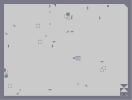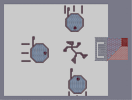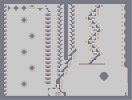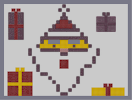Stop Following Me! I'm a Slow Learner To Bad JOY 8-Bit Santa

## Comments

Pages: (0)

### Just

plain awesome. I saw this before I made an account, and I thought it was the best ^_^

5aved

5aved

### the laser drone as rudolph

makes me laughing^^
nice work, 5aved
reindeersdrones!!!
santa money!!!

good times
5aved.

5aved

### Well...

...it's actually pretty sweet.
LMAO

5/5!

### yea this was my 3rd n art

and it was a year ago, i promise that my new arts will be better

### If the ninja was more visible

this would've been perfect.

The third 4 in a row I've given to one of your maps :P

### this is awesome

but I mistaken'd santa's sack to be santa and I can barely see the ninja

### thats awesome!!!!!

like rudolf and "saNta's" sack of gold

### I like this.

It truly is amazing what one can do with the limits of N to make N art!

As for you're last comment on Pi [nmaps.net], I don't really understand the last part, especially the second-to-last sentence; If you could send a clearer message for the sake of criticism, it would be much appreciated.

4/5aved

### 2gt

my demor
Demo Data 466:0|0|0|0|0|17895424|17895697|17895697|17895697|71582797|71582788|17895765|17895697|17895697|1118481|17825792|17895697|17895697|17895697|17895697|17895697|17895697|72089873|71582788|107374182|107374182|107374182|22369604|17895697|1118481|79691776|107365444|107374182|107374182|73819750|68|0|0|33554432|546|107266048|107374182|107374182|107374182|107374182|35791394|115483170|17891398|17895697|17895697|17895697|0|0|0|89478476|89478485|89478485|89478485|70997|35790848|35791394|35791394|107405858|107374182|107374182|107374182|26214

### omg

ddue i'm rellay high,.,,,, on paper wih paper in it! I think, or maaybe ait's the water, bbut dangm this thing alooks kinda coal
:D How cute!

### Pretty

3.5 for the effort, 0.5 for the results.

rudolf!

rudolf!

Drone-deer
N-ta claus
xD
/end lame names

It's pretty good

### yea

the ninja was what i couln't get right so finally i just gave up and it what u see now

### :D

I like it, but the ninja/Santa isn't done so well. It's still fun to look at, though.